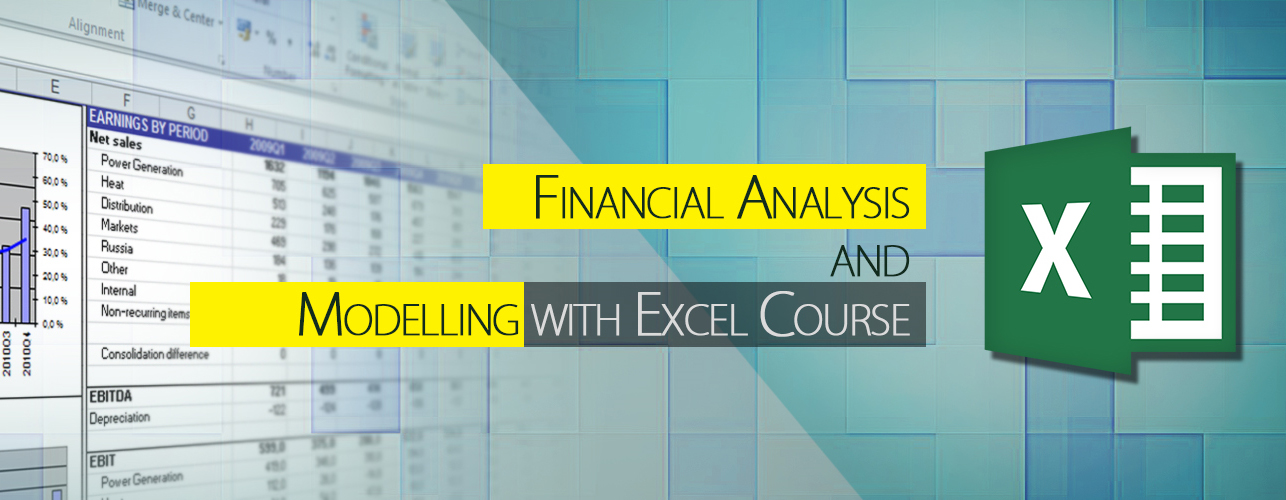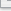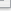# Financial Analysis and Modelling with ExcelThe Financial Analysis and Modelling with Excel training course is aimed to equip students with computation skills to analyse and evaluate projects, budgets and other finance related activities on their suitability for investment. With the help of the Excel’s business data analysis tools, financial models can be constructed to calculate, forecast, estimate financial numbers which can help companies to allocate resources and evaluate potential projects for maximum return-on-investment.

The course will cover Excel financial functions, Excel’s business modelling tools, forecasting techniques, financial statement analysis tools, time value of money and more. As there is no simply way to learn without doing it, students will have extensive practice chance to work on the easy to follow exercises.

The daytime or evening classes are offered to match students’ different training needs. If you are business owners, finance and accounting professionals, business analyst, consultants or individuals for personal finance will find that course useful.# What you’ll learn

## Building Financial Formulas

• Working with Interest Rates
• Calculating the Future Value
• Calculating the Present Value
• Calculating Net Present Value
• Discounting Cash Flows
• Calculating the Payback Period

• Using What-If Analysis
• Working with Goal Seek
• Working with Scenarios

## Financial Statement Analysis Tools

• Ratio Analysis
• Analyzing Liquidity Ratios
• Analyzing Profitability Ratios
• Leverage Ratios
• Activity Ratios

## Time Value of Money

• Single Cash Flow
• Annuity
• NPV Using Constant Discounting
• NPV Using General Discounting
• Loan Amortization
• Sensitivity AnalysisSCHEDULESFAM2081 - 廣東話 01 AugFAM2082 - 廣東話 16 AugFAM2083 - 廣東話 30 AugFAM2091 - 廣東話 28 SepFAM2092 - 廣東話 29 SepRELATING COURSES## Forecasting with Moving Average and Chart

• Creating Moving Average Forecasts with Excel’s Charts
• Analyzing Trends Using Best-Fit Lines
• Plotting a Best-Fit Trendlines

## Forcasting on Linear Data

• Understanding the Regression Equation
• Understanding R2 - coefficient of determination
• Making Linear Forecasts: The TREND and LINEST Function

## Forcasting on Nonlinear Data

• Working with an Exponential Trend
• Working with a LogarithmicTrend
• Making Nonlinear Forecasts: The GROWTH Function
• Working with a PowerTrend
• Using Polynomial Regression Analysis
• Using Mulitple Regression Analysis

## Building Capital Budgeting Models

• Forecasting Cash Flows
• Working Capital
• Sensitivity Analysis
• Break-Even Analysis on accounting profit
• Break-Even Analysis on NPV